# Recurrent Neural Networks¶

Last time, we began tackling the problem of predicting the sentiment of tweets based on its text. We used GloVe embeddings, and summed up the embedding of each word in a tweet to obtain a representation of the tweet. We then built a model to predict the tweet's sentiment based on its representation.

One of the drawbacks of the previous approach is that the order of words is lost. The tweets "the cat likes the dog" and "the dog likes the cat" would have the exact same embedding, even though the sentences have different meanings.

Today, we wil use a recurrent neural network. We will treat each tweet as a sequence of words. Like before, we will use GloVe embeddings as inputs to the recurrent network. (As a sidenote, not all recurrent neural networks use word embeddings as input. If we had a small enough vocabulary, we could have used a one-hot embedding of the words.)In :
import csv
import torch
import torch.nn as nn
import torch.nn.functional as F
import torch.optim as optim
import torchtext
import numpy as np
import matplotlib.pyplot as plt

def get_data():
return csv.reader(open("training.1600000.processed.noemoticon.csv", "rt", encoding="latin-1"))

def split_tweet(tweet):
# separate punctuations
tweet = tweet.replace(".", " . ") \
.replace(",", " , ") \
.replace(";", " ; ") \
.replace("?", " ? ")
return tweet.split()

glove = torchtext.vocab.GloVe(name="6B", dim=50, max_vectors=10000) # use 10k most common words


Since we are going to store the individual words in a tweet, we will defer looking up the word embeddings. Instead, we will store the index of each word in a PyTorch tensor.

In :
def get_tweet_words(glove_vector):
train, valid, test = [], [], []
for i, line in enumerate(get_data()):
if i % 29 == 0:
tweet = line[-1]
idxs = [glove_vector.stoi[w]        # lookup the index of word
for w in split_tweet(tweet)
if w in glove_vector.stoi] # keep words that has an embedding
if not idxs: # ignore tweets without any word with an embedding
continue
idxs = torch.tensor(idxs) # convert list to pytorch tensor
label = torch.tensor(int(line == "4")).long()
if i % 5 < 3:
train.append((idxs, label))
elif i % 5 == 4:
valid.append((idxs, label))
else:
test.append((idxs, label))
return train, valid, test

train, valid, test = get_tweet_words(glove)


Here's what an element of the training set looks like:

In :
tweet, label = train
print(tweet)
print(label)

tensor([  2,  11,   1,   7,   2, 405,   3,   4,  88,  20,   2,  89])
tensor(0)


Unlike in the past, each element of the training set will have a different shape. The difference will present some difficulties when we discuss batching later on.

In :
for i in range(10):
tweet, label = train[i]
print(tweet.shape)

torch.Size()
torch.Size()
torch.Size()
torch.Size()
torch.Size()
torch.Size()
torch.Size()
torch.Size()
torch.Size()
torch.Size()


## Embedding¶

We are also going to use an nn.Embedding layer, instead of using the variable glove directly. The reason is that the nn.Embedding layer lets us look up the embeddings of multiple words simultaneously.

In :
glove_emb = nn.Embedding.from_pretrained(glove.vectors)

# Example: we use the forward function of glove_emb to lookup the
# embedding of each word in tweet
tweet_emb = glove_emb(tweet)
tweet_emb.shape

Out:
torch.Size([28, 50])

## Recurrent Neural Network Module¶

PyTorch has variations of recurrent neural network modules. These modules computes the following:

$$hidden = f(lasthidden, input)$$ $$output = g(hidden)$$

They are more complex than the usual neural networks layers, so let's take a look:

In :
rnn_layer = nn.RNN(input_size=50,    # dimension of the input repr
hidden_size=50,   # dimension of the hidden units
batch_first=True) # input format is [batch_size, seq_len, repr_dim]


Now, let's try and run this untrained rnn_layer on tweet_emb. We will need to add an extra dimension to tweet_emb to account for batching. We will also need to initialize a set of hidden units of size [batch_size, 1, repr_dim], to be used for the first set of computations.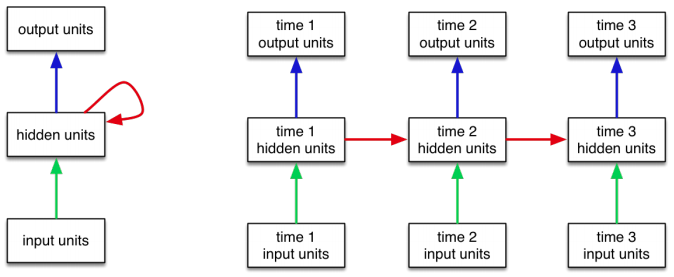In :
tweet_input = tweet_emb.unsqueeze(0) # add the batch_size dimension
h0 = torch.zeros(1, 1, 50)     # initial hidden layer
out, last_hidden = rnn_layer(tweet_input, h0)


Now, let's look at the output and hidden dimensions that we have:

In :
print(out.shape)
print(last_hidden.shape)

torch.Size([1, 28, 50])
torch.Size([1, 1, 50])


The shape of the hidden units is the same as our initial h0. The variable out, though, has the same shape as our input. The variable contains the concatenation of all of the output units for each word (i.e. at each time point).

Normally, we only care about the output at the final time point, which we can extract like this.

In :
out[:,-1,:]

Out:
tensor([[ 0.5087, -0.2452, -0.3332, -0.6969,  0.0115, -0.3327, -0.4505, -0.1720,
0.3529,  0.3845,  0.5768,  0.1423,  0.0987,  0.0124,  0.4015,  0.2342,
0.3808, -0.4786,  0.2799,  0.0968, -0.0522, -0.1727,  0.4170,  0.3139,
-0.2475,  0.0683, -0.0069,  0.2908, -0.1177,  0.2518, -0.5535, -0.1260,
-0.4213,  0.2321, -0.0207, -0.3354,  0.5181, -0.7444, -0.3865,  0.6986,
0.2458, -0.5219, -0.1450, -0.3218,  0.2388,  0.2452,  0.6348, -0.2476,
-0.0486,  0.3465]], grad_fn=<SelectBackward>)

This tensor summarizes the entire tweet, and can be used as an input to a classifier.

## Building a Model¶

Let's put both the embedding layer, the RNN and the classifier into one model:

In :
class TweetRNN(nn.Module):
def __init__(self, input_size, hidden_size, num_classes):
super(TweetRNN, self).__init__()
self.emb = nn.Embedding.from_pretrained(glove.vectors)
self.hidden_size = hidden_size
self.rnn = nn.RNN(input_size, hidden_size, batch_first=True)
self.fc = nn.Linear(hidden_size, num_classes)

def forward(self, x):
# Look up the embedding
x = self.emb(x)
# Set an initial hidden state
h0 = torch.zeros(1, x.size(0), self.hidden_size)
# Forward propagate the RNN
out, _ = self.rnn(x, h0)
# Pass the output of the last time step to the classifier
out = self.fc(out[:, -1, :])
return out

model = TweetRNN(50, 50, 2)


Now, this model has a very similar API as the previous. We should be able to train this model similar to any other model that we have trained before. However, there is one caveat that we have been avoiding this entire time, batching.

## Batching¶

Unfortunately, we will not be able to use DataLoader with a batch_size of greater than one. This is because each tweet has a different shaped tensor.

In :
for i in range(10):
tweet, label = train[i]
print(tweet.shape)

torch.Size()
torch.Size()
torch.Size()
torch.Size()
torch.Size()
torch.Size()
torch.Size()
torch.Size()
torch.Size()
torch.Size()


PyTorch implementation of DataLoader class expects all data samples to have the same shape. So, if we create a DataLoader like below, it will throw an error when we try to iterate over its elements.

In :
#will_fail = torch.utils.data.DataLoader(train, batch_size=128)
#for elt in will_fail:
#    print("ok")


So, we will need a different way of batching.

One strategy is to pad shorter sequences with zero inputs, so that every sequence is the same length. The following PyTorch utilities are helpful.

• torch.nn.utils.rnn.pad_sequence
• torch.nn.utils.rnn.pad_packed_sequence
• torch.nn.utils.rnn.pack_sequence
• torch.nn.utils.rnn.pack_padded_sequence
In :
from torch.nn.utils.rnn import pad_sequence

tweet_padded = pad_sequence([tweet for tweet, label in train[:10]],
batch_first=True)

Out:
torch.Size([10, 28])

This way, we can pass multiple tweets through the RNN at once!

In :
out = model(tweet_padded)
out.shape

Out:
torch.Size([10, 2])

One issue we overlooked was that in our TweetRNN model, we always take the last output unit as input to the final classifier. Now that we are padding the input sequences, we should really be using the output at a previous time step. Recurrent neural networks therefore require much more record keeping than MLPs or even CNNs.

There is yet another problem: the longest tweet has many, many more words than the shortest. Padding tweets so that every tweet has the same length as the longest tweet is impractical. Padding tweets in a mini-batch, however, is much more reasonable.

In practice, practitioners will batch together tweets with the same length. For simplicity, we will do the same. We will implement a (more or less) straightforward way to batch tweets. Our implementation will be flawed, and we will discuss these flaws.

In :
import random

class TweetBatcher:
def __init__(self, tweets, batch_size=32, drop_last=False):
# store tweets by length
self.tweets_by_length = {}
for words, label in tweets:
# compute the length of the tweet
wlen = words.shape
# put the tweet in the correct key inside self.tweet_by_length
if wlen not in self.tweets_by_length:
self.tweets_by_length[wlen] = []
self.tweets_by_length[wlen].append((words, label),)

#  create a DataLoader for each set of tweets of the same length
tweets,
batch_size=batch_size,
shuffle=True,
drop_last=drop_last) # omit last batch if smaller than batch_size
for wlen, tweets in self.tweets_by_length.items()}

def __iter__(self): # called by Python to create an iterator
# make an iterator for every tweet length
while iters:
# pick an iterator (a length)
im = random.choice(iters)
try:
yield next(im)
except StopIteration:
# no more elements in the iterator, remove it
iters.remove(im)


Let's take a look at our batcher in action. We will set drop_last to be true for training, so that all of our batches have exactly the same size.

In :
for i, (tweets, labels) in enumerate(TweetBatcher(train, drop_last=True)):
if i > 5: break
print(tweets.shape, labels.shape)

torch.Size([32, 3]) torch.Size()
torch.Size([32, 24]) torch.Size()
torch.Size([32, 20]) torch.Size()
torch.Size([32, 17]) torch.Size()
torch.Size([32, 23]) torch.Size()
torch.Size([32, 10]) torch.Size()


Just to verify that our batching is reasonable, here is a modification of the get_accuracy function we wrote last time.

In :
def get_accuracy(model, data_loader):
correct, total = 0, 0
for tweets, labels in data_loader:
output = model(tweets)
pred = output.max(1, keepdim=True)
correct += pred.eq(labels.view_as(pred)).sum().item()
total += labels.shape
return correct / total

test_loader = TweetBatcher(test, batch_size=64, drop_last=False)

Out:
0.49012334229806176

Our training code will also be very similar to the code we wrote last time:

In :
def train_rnn_network(model, train, valid, num_epochs=5, learning_rate=1e-5):
criterion = nn.CrossEntropyLoss()
optimizer = torch.optim.Adam(model.parameters(), lr=learning_rate)
losses, train_acc, valid_acc = [], [], []
epochs = []
for epoch in range(num_epochs):
for tweets, labels in train:
pred = model(tweets)
loss = criterion(pred, labels)
loss.backward()
optimizer.step()
losses.append(float(loss))

epochs.append(epoch)
print("Epoch %d; Loss %f; Train Acc %f; Val Acc %f" % (
epoch+1, loss, train_acc[-1], valid_acc[-1]))
# plotting
plt.title("Training Curve")
plt.plot(losses, label="Train")
plt.xlabel("Epoch")
plt.ylabel("Loss")
plt.show()

plt.title("Training Curve")
plt.plot(epochs, train_acc, label="Train")
plt.plot(epochs, valid_acc, label="Validation")
plt.xlabel("Epoch")
plt.ylabel("Accuracy")
plt.legend(loc='best')
plt.show()


Let's train our model. Note that there will be some inaccuracies in computing the training loss. We are dropping some data from the training set by setting drop_last=True. Again, the choice is not ideal, but simplifies our code.

In :
model = TweetRNN(50, 50, 2)
train_loader = TweetBatcher(train, batch_size=64, drop_last=True)
valid_loader = TweetBatcher(valid, batch_size=64, drop_last=False)

Epoch 1; Loss 0.699729; Train Acc 0.639347; Val Acc 0.641733
Epoch 2; Loss 0.642706; Train Acc 0.653534; Val Acc 0.652101
Epoch 3; Loss 0.630883; Train Acc 0.655355; Val Acc 0.647658
Epoch 4; Loss 0.615876; Train Acc 0.656665; Val Acc 0.650898
Epoch 5; Loss 0.606689; Train Acc 0.658966; Val Acc 0.653305
Epoch 6; Loss 0.575744; Train Acc 0.662641; Val Acc 0.655249
Epoch 7; Loss 0.581709; Train Acc 0.662321; Val Acc 0.656175
Epoch 8; Loss 0.588590; Train Acc 0.664015; Val Acc 0.657193
Epoch 9; Loss 0.616296; Train Acc 0.665069; Val Acc 0.658304
Epoch 10; Loss 0.547985; Train Acc 0.668616; Val Acc 0.660711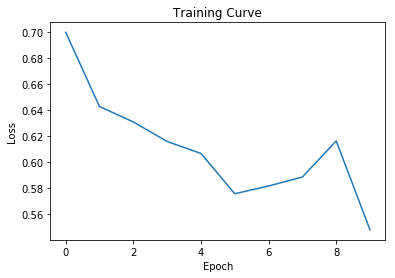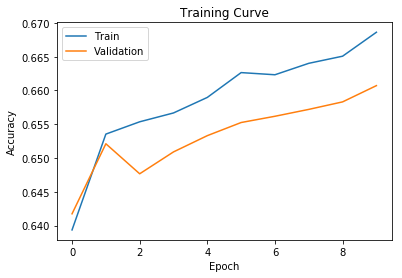Out:
0.6537141797273486

The hidden size and the input embedding size don't have to be the same.

In :
#model = TweetRNN(50, 100, 2)


## LSTM for Long-Term Dependencies¶

There are variations of RNN's that are more powerful. One such variation is the Long Short-Term Memory module. An LSTM is like a more powerful version of an RNN that is better at perpetuating long-term dependencies. Instead of having only one hidden state, an LSTM keeps track of both a hidden state and a cell state.

In :
lstm_layer = nn.LSTM(input_size=50,   # dimension of the input repr
hidden_size=50,   # dimension of the hidden units
batch_first=True) # input format is [batch_size, seq_len, repr_dim]


Remember the single tweet that we worked with earlier?

In :
tweet_emb.shape

Out:
torch.Size([28, 50])

This is how we can feed this tweet into the LSTM, similar to what we tried with the RNN earlier.

In :
tweet_input = tweet_emb.unsqueeze(0) # add the batch_size dimension
h0 = torch.zeros(1, 1, 50)     # initial hidden layer
c0 = torch.zeros(1, 1, 50)     # initial cell state
out, last_hidden = lstm_layer(tweet_input, (h0, c0))
out.shape

Out:
torch.Size([1, 28, 50])

So an LSTM version of our model would look like this:

In :
class TweetLSTM(nn.Module):
def __init__(self, input_size, hidden_size, num_classes):
super(TweetLSTM, self).__init__()
self.emb = nn.Embedding.from_pretrained(glove.vectors)
self.hidden_size = hidden_size
self.rnn = nn.LSTM(input_size, hidden_size, batch_first=True)
self.fc = nn.Linear(hidden_size, num_classes)

def forward(self, x):
# Look up the embedding
x = self.emb(x)
# Set an initial hidden state and cell state
h0 = torch.zeros(1, x.size(0), self.hidden_size)
c0 = torch.zeros(1, x.size(0), self.hidden_size)
# Forward propagate the LSTM
out, _ = self.rnn(x, (h0, c0))
# Pass the output of the last time step to the classifier
out = self.fc(out[:, -1, :])
return out

model_lstm = TweetLSTM(50, 50, 2)

Epoch 1; Loss 0.662832; Train Acc 0.669447; Val Acc 0.658674
Epoch 2; Loss 0.527028; Train Acc 0.664430; Val Acc 0.659322
Epoch 3; Loss 0.679501; Train Acc 0.671971; Val Acc 0.663118
Epoch 4; Loss 0.589477; Train Acc 0.666475; Val Acc 0.659507
Epoch 5; Loss 0.566892; Train Acc 0.671875; Val Acc 0.660063
Epoch 6; Loss 0.670599; Train Acc 0.654652; Val Acc 0.648491
Epoch 7; Loss 0.564536; Train Acc 0.676572; Val Acc 0.667562
Epoch 8; Loss 0.578968; Train Acc 0.674016; Val Acc 0.661544
Epoch 9; Loss 0.633945; Train Acc 0.679863; Val Acc 0.670802
Epoch 10; Loss 0.558089; Train Acc 0.680374; Val Acc 0.672931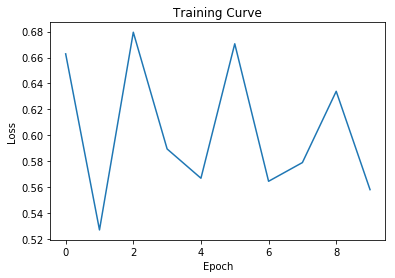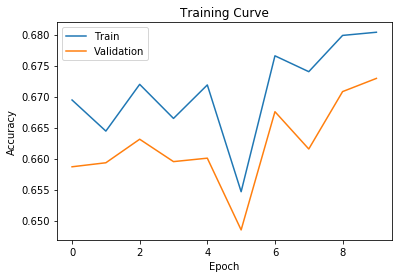Out:
0.6685523509227488